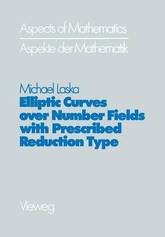Image credit: dr. shordzi, on Flickr
Filter
(found 1 product)Let K be an algebraic number field. The function attaching to each elliptic curve over K its conductor is constant on isoger. y classes of elliptic curves over K (for the definitions see chapter 1). Ioreover, for a given ideal a in OK the number of isogeny classes of elliptic ...
Elliptic Curves Over Number Fields with Prescribed Reduction Type
Let K be an algebraic number field. The function attaching to each elliptic curve over K its conductor is constant on isoger. y classes of elliptic curves over K (for the definitions see chapter 1). Ioreover, for a given ideal a in OK the number of isogeny classes of elliptic curves over K with conductor a is finite. In these notes we deal with the following problem: How can one explicitly construct a set of representatives for the isogeny classes of elliptic curves over K with conductor a for a given ideal a in OK? The conductor of an elliptic curve over K is a numerical invariant which measures, in some sense, the badness of the reduction of the elliptic curve modulo the prime ideals in OK' It plays an important role in the famous Weil-Langlands conjecture on the connection between elliptic curves over K and congruence subgroups in 5L2(OK) - In case K this connection can be stated as follows. For any ideal a = (N) in let ro(N) be the congruence subgroup ro(N) { (: ) E 5L2 ( ) c E (N) } of 5L2 ( ) and let 52 (fo (N be the space of cusp forms of weight 2 for r 0 (N) Now Weil conjectured that there exists a bijection between the rational normalized eigenforms in 52(ro(N for the Heckealgebra and the - 2 - Lsug ny classes uf elliptic curves over with conductor a = (N) .